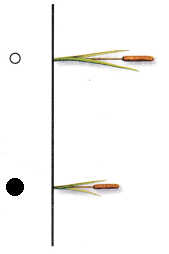# Texas Go Math Kindergarten Lesson 19.2 Answer Key Compare Lengths

Refer to our Texas Go Math Kindergarten Answer Key Pdf to score good marks in the exams. Test yourself by practicing the problems from Texas Go Math Kindergarten Lesson 19.2 Answer Key Compare Lengths.

## Texas Go Math Kindergarten Lesson 19.2 Answer Key Compare Lengths

Essential Question
How can you compare the lengths of two objects?

Explore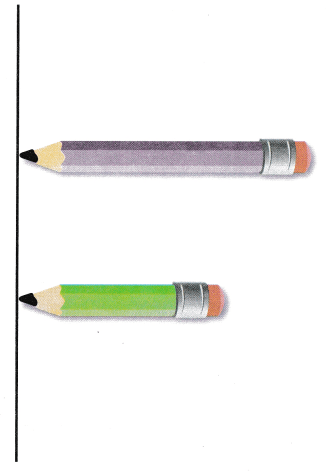Explanation:
Circled the longer pencil
and crossed the shorter pencil.

Directions
Look at the pencils. Compare the lengths of the two pencils. Use the words longer than, shorter than, or about the some length to describe the lengths. Draw around the longer pencil. Draw on X on the shorter pencil.

Share and Show

Question 1.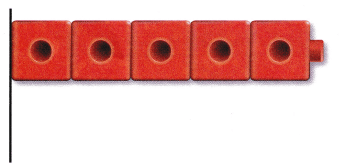Explanation:
A longer orange cube is drawn than the shorter red cube

Question 2.Explanation:
A longer orange cube is drawn than the shorter green cube

Question 3.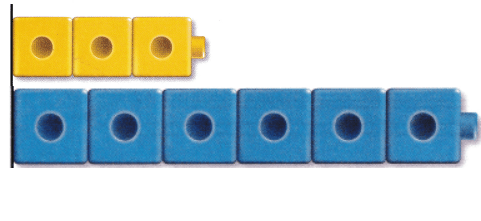Explanation:
A longer blue cube is drawn than the shorter yellow cube

Directions 1-3. Make a cube train that is longer than the cube train shown. Draw and color the cube train.

Question 4.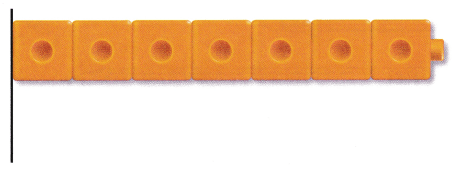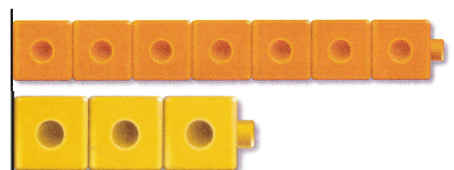Explanation:
Short yellow cube is drawn than the longer yellow cube.

Question 5.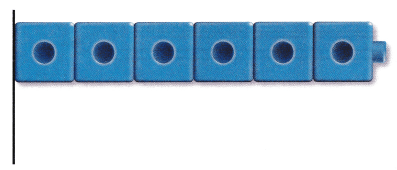Explanation:
Short yellow cube is drawn than the longer blue cube.

Question 6.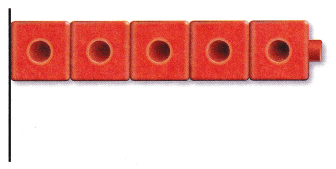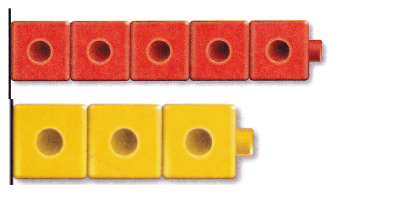Explanation:
Short yellow cube is drawn than the longer red cube.

Directions
4-6. Make a cube train that is shorter than the cube train shown. Draw and color the cube train.

Problem Solving

Question 7.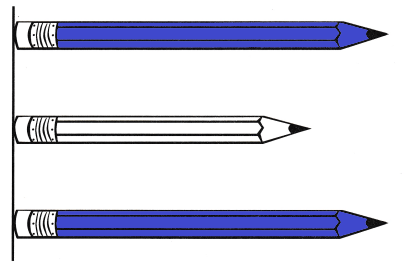Explanation:
The blue pencils are in same length
The same length pencils are colored with color blue.

Question 8.Explanation:
The purple crayon is shorter than the orange crayon
so, marked the purple crayon.

Directions
7. Two of these pencils are about the some length. Color those pencils. 8. Choose the correct answer. Which crayon is shorter?

Home Activity

• Show your child a pencil and ask him or her to find an object that is longer than the pencil. Repeat with an object that is shorter than the pencil and one that is about the some length.

### Texas Go Math Kindergarten Lesson 19.2 Homework and Practice

Question 1.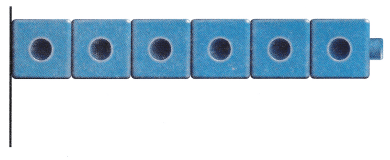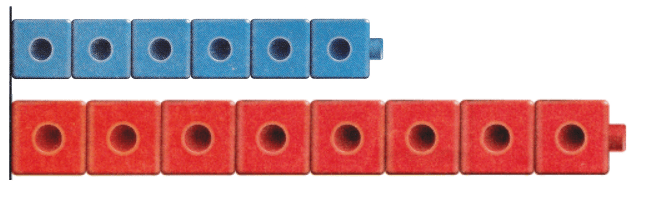Explanation:
Drawn a longer cube than the given short blue cube
so, drawn a longer red cube.

Question 2.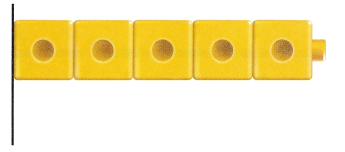Explanation:
Drawn a longer cube than the given short yellow cube
so, drawn a longer red cube.

Question 3.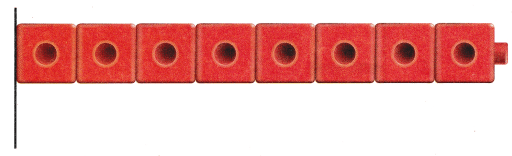Explanation:
Drawn a shorter cube
the given red cube is longer so, drawn a shorter cube.

Directions 1-2. Draw and color a cube train that s longer than the cube train shown. 3. Draw and color a cube train that is shorter than the cube train shown.

Texas Test Prep

Question 4.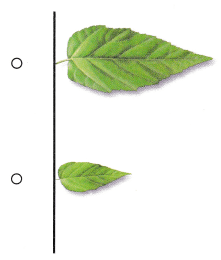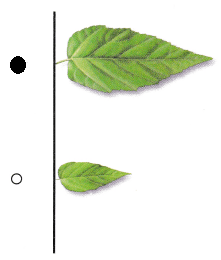Explanation:
Marked the longer leaf
first leaf is longer than the second leaf.

Question 5.